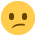# I got the following question in a WKAF mock exam and I can't work it out...

Department 4 & 5 have combined sales figures of £459,051.
Dept. 4 made twice as many sales as Dept. 5. What is the figure for sales made by Dept. 4?

• This can be solved with some basic algebra.

x = department 5 sales.
Department 4 sales therefore = 2x (twice as many as department 5)
Total sales = x + 2x = 3x

Therefore 3x = 459,051
And x = 153,017

Department 4 = 2x = 2 x 153,017 = 306,034
• @CeeJaySix Well, that's embarrassingThanks so much for your help!